Courses

NCERT Solutions, Pair Of Linear Equations In Two Variables, Class 10, Maths Notes - Class 10

Class 10: NCERT Solutions, Pair Of Linear Equations In Two Variables, Class 10, Maths Notes - Class 10

The document NCERT Solutions, Pair Of Linear Equations In Two Variables, Class 10, Maths Notes - Class 10 is a part of Class 10 category.
All you need of Class 10 at this link: Class 10

Page No: 44

Exercise 3.1

1. Aftab tells his daughter, "Seven years ago, I was seven times as old as you were then. Also, three years from now, I shall be three times as old as you will be." (Isn't this interesting?) Represent this situation algebraically and graphically.

Let present age of Aftab be x
And, present age of daughter is represented by y
Then Seven years ago,
Age of Aftab = -7
Age of daughter = y-7
According to the question,
(- 7)  = 7 (– 7 )
– 7 = 7 – 49
x- 7 = - 49 7
– 7y = - 42 …(i)
x = 7y – 42
Putting y = 5, 6 and 7, we get
x = 7 × 5 - 42 = 35 - 42 = - 7
x = 7 × 6 - 42 = 42 – 42 = 0
x = 7 × 7 – 42 = 49 – 42 = 7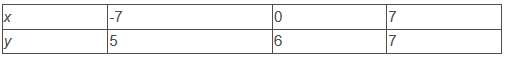Three years from now ,
Age of Aftab = 3
Age of daughter = 3
According to the question,
( 3) = 3 ( 3)
3 = 3 9
-3= 9-3
-3 = 6 …(ii)
= 3 6
Putting, = -2,-1 and 0, we get
= 3 × - 2 6 = -6 6 =0
= 3 × - 1 6 = -3 6 = 3
= 3 × 0 6 = 0 6 = 6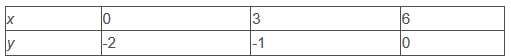Algebraic representation
From equation (i) and (ii)
– 7 = – 42 …(i)
- 3 = 6 …(ii)
Graphical representation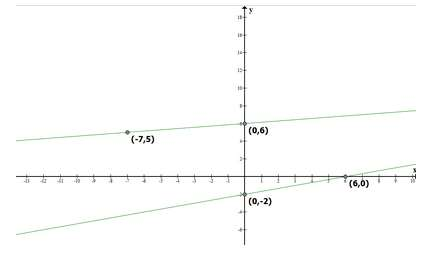2. The coach of a cricket team buys 3 bats and 6 balls for Rs 3900. Later, she buys another bat and 3 more balls of the same kind for Rs 1300. Represent this situation algebraically and geometrically.

Let cost of one bat = Rs x
Cost of one ball = Rs y
3 bats and 6 balls for Rs 3900 So that
3 6y = 3900 … (i)
Dividing equation by 3, we get
2y = 1300
Subtracting 2y both side we get
x = 1300 – 2
Putting y = -1300, 0 and 1300 we get
x = 1300 – 2 (-1300) = 1300 2600 = 3900
= 1300 -2(0) = 1300 - 0 = 1300
x = 1300 – 2(1300) = 1300 – 2600 = - 1300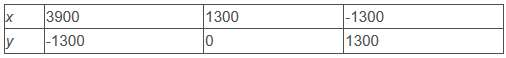Given that she buys another bat and 2 more balls of the same kind for Rs 1300
So, we get
x 2= 1300 … (ii)
Subtracting 2y both side we get
= 1300 – 2y
Putting y = - 1300, 0 and 1300 we get
x = 1300 – 2 (-1300) = 1300 2600 = 3900
= 1300 – 2 (0) = 1300 - 0 = 1300
= 1300 – 2(1300) = 1300 – 2600 = -1300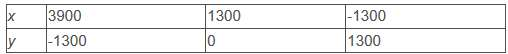Algebraic representation
3 6y = 3900 … (i)
2 = 1300 … (ii)
Graphical representation,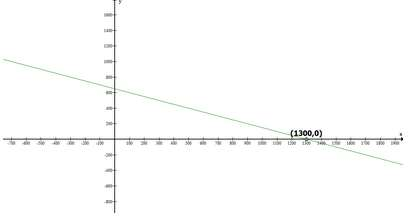3. The cost of 2 kg of apples and 1kg of grapes on a day was found to be Rs 160. After a month, the cost of 4 kg of apples and 2 kg of grapes is Rs 300. Represent the situation algebraically and geometrically.

Let cost each kg of apples = Rs x
Cost of each kg of grapes = Rs y
Given that the cost of 2 kg of apples and 1kg of grapes on a day was found to be Rs 160
So that
2 = 160 … (i)
2x = 160 - y
x = (160 – y)/2
Let y = 0 , 80 and 160,  we get
x = (160 – ( 0 )/2 = 80
x = (160- 80 )/2 = 40
x = (160 – 2 × 80)/2 = 0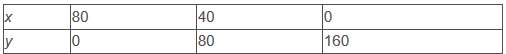Given that the cost of 4 kg of apples and 2 kg of grapes is Rs 300
So we get
4x 2= 300 … (ii)
Dividing by 2 we get
2x y = 150
Subtracting 2x both side, we get
= 150 – 2x
Putting x = 0 , 50 , 100 we get
= 150 – 2 × 0 = 150
= 150 – 2 ×  50 = 50
= 150 – 2 × (100) = -50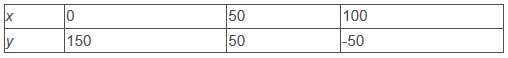Algebraic representation,
2 y = 160 … (i)
4x 2y = 300 … (ii)

Graphical representation,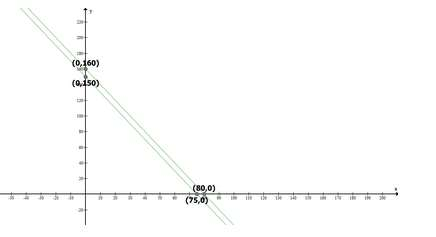Page No: 49

Exercise 3.2

1. Form the pair of linear equations in the following problems, and find their solutions graphically.

(i) 10 students of Class X took part in a Mathematics quiz. If the number of girls is 4 more than the number of boys, find the number of boys and girls who took part in the quiz.

Let number of boys = x
Number of girls = y
Given that total number of student is 10 so that
= 10
Subtract y both side we get
= 10 – y
Putting = 0 , 5, 10 we get
= 10 – 0 = 10
= 10 – 5 = 5
= 10 – 10 = 0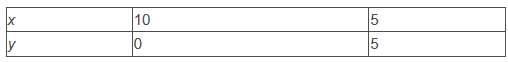Given that If the number of girls is 4 more than the number of boys
So that
= 4
Putting x = -4, 0, 4, and we get
= - 4 4 = 0
= 0 4 = 4
= 4 4 = 8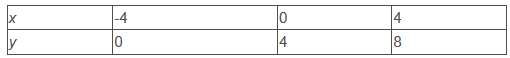Graphical representation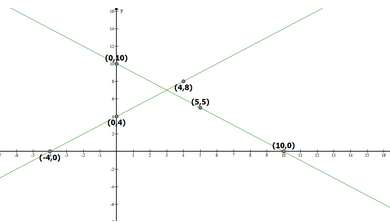Therefore, number of boys = 3 and number of girls = 7.

(ii) 5 pencils and 7 pens together cost Rs 50, whereas 7 pencils and 5 pens together cost Rs 46. Find the cost of one pencil and that of one pen.

Let cost of pencil = Rs x
Cost of pens = Rs y
5 pencils and 7 pens together cost Rs 50,
So we get
5x 7y = 50
Subtracting 7y both sides we get
5x = 50 – 7y
Dividing by 5 we get
x = 10 - 7 y /5
Putting value of y = 5 , 10 and 15 we get
x = 10 – 7 × 5/5 = 10 – 7 = 3
x = 10 – 7 × 10/5 = 10 – 14 = - 4

x = 10 – 7 × 15/5 = 10 – 21 = - 11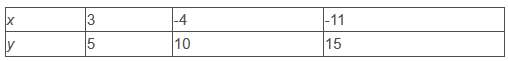Given that 7 pencils and 5 pens together cost Rs 46
7x 5y = 46
Subtracting 7x both side we get
5y = 46 – 7x
Dividing by 5 we get
y = 46/5 - 7x/5
y = 9.2 – 1.4x
Putting x = 0 , 2 and 4 we get
y = 9.2 – 1.4 × 0 = 9.2 – 0 = 9.2
y = 9.2 – 1.4 (2) = 9.2 – 2.8 = 6.4
y = 9.2 – 1.4 (4) = 9.2 – 5.6 = 3.6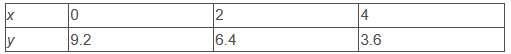Graphical representation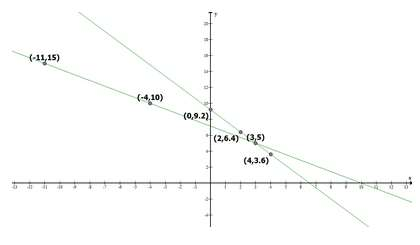Therefore, cost of one pencil = Rs 3 and cost of one pen = Rs 5.

2. On comparing the ratios a1/a2 , b1/b2 and c1/c2, find out whether the lines representing the following pairs of linear equations intersect at a point, are parallel or coincident.

(i) 5x – 4y 8 = 0
7x 6y – 9 = 0

Comparing these equation with
a1x  b1y c1 = 0
a2x  b2y  c2= 0

We get
a1 = 5, b1 = -4, and c1 = 8
a2 =7, b2 = 6 and c2 = -9
a1/a2 = 5/7,
b1/b2 = -4/6 and
c1/c2 = 8/-9
Hence, a1/a2 ≠ b1/b2

Therefore, both are intersecting lines at one point.

(ii) 9x 3y 12 = 0
18x 6y 24 = 0
Comparing these equations with

a1x  b1y c1 = 0

a2x  b2y  c2= 0
We get
a1 = 9, b1 = 3, and c1 = 12
a2 = 18, b2 = 6 and c2 = 24
a1/a2 = 9/18 = 1/2
b1/b2 = 3/6 = 1/2 and
c1/c2 = 12/24 = 1/2
Hence, a1/a2b1/b= c1/c2

Therefore, both lines are coincident

(iii) 6x – 3y 10 = 0
2xy 9 = 0
Comparing these equations with

a1x   b1y   c1 = 0

a2x   b2y   c2= 0

We get
a1 = 6, b1 = -3, and c1 = 10
a2 = 2, b2 = -1 and c2 = 9
a1/a2 = 6/2 = 3/1
b1/b2 = -3/-1 = 3/1 and
c1/c2 = 12/24 = 1/2
Hence, a1/a2b1/b c1/c2

Therefore, both lines are parallel

3. On comparing the ratios a1/a2 , b1/b2 and c1/c2 find out whether the following pair of linear equations are consistent, or inconsistent.
(i) 3x 2y = 5 ; 2x – 3y = 7
(ii) 2x – 3y = 8 ; 4x – 6y = 9
(iii) 3/2x 5/3y = 7 ; 9– 10y = 14
(iv) 5x – 3y = 11 ; – 10x 6y = –22
(v) 4/3x 2y =8 ; 2x 3y = 12

(i) 3x 2y = 5 ; 2x – 3y = 7
a1/a2 = 3/2
b1/b2 = -2/3 and
c1/c2 = 5/7
Hence, a1/a2 ≠ b1/b2
These linear equations are intersecting each other at one point and thus have only one possible solution. Hence, the pair of linear equations is consistent.

(ii) 2x – 3y = 8 ; 4x – 6y = 9
a1/a2 = 2/4 = 1/2
b1/b2 = -3/-6 = 1/2 and
c1/c2 = 8/9
Hence, a1/a2b1/b c1/c2

Therefore, these linear equations are parallel to each other and thus have no possible solution. Hence, the pair of linear equations is inconsistent.

(iii) 3/2x  5/3y = 7 ; 9– 10y = 14
a1/a2 = 3/2/9 = 1/6
b1/b2 = 5/3/-10 = -1/6 and
c1/c2 = 7/14 = 1/2
Hence, a1/a2 ≠ b1/b2

Therefore, these linear equations are intersecting each other at one point and thus have only one possible solution. Hence, the pair of linear equations is consistent.

(iv) 5x – 3y = 11 ; – 10x  6y = –22
a1/a2 = 5/-10 = -1/2
b1/b2 = -3/6 = -1/2 and
c1/c2 = 11/-22 = -1/2
Hence, a1/a2b1/b2 = c1/c2

Therefore, these linear equations are coincident pair of lines and thus have infinite number of possible solutions. Hence, the pair of linear equations is consistent.

(v) 4/3x  2y =8 ; 2x  3y = 12
a1/a2 = 4/3/2 = 2/3
b1/b2 = /3 and
c1/c2 = 8/12 = 2/3
Hence, a1/a2b1/b2 = c1/c2

Therefore, these linear equations are coincident pair of lines and thus have infinite number of possible solutions. Hence, the pair of linear equations is consistent.

4. Which of the following pairs of linear equations are consistent/inconsistent? If consistent, obtain the solution graphically:
(i) x y = 5, 2x 2y = 10

(ii) xy = 8, 3x – 3y = 16

(iii) 2x y – 6 = 0, 4x – 2y – 4 = 0

(iv) 2x – 2y – 2 = 0, 4x – 4y – 5 = 0

(i) x   y = 5; 2x  2y = 10
a1/a2 = 1/2
b1/b2 = 1/2 and
c1/c2 = 5/10 = 1/2
Hence, a1/a2b1/b2 = c1/c2
Therefore, these linear equations are coincident pair of lines and thus have infinite number of possible solutions. Hence, the pair of linear equations is consistent.

x y = 5

x = 5 - y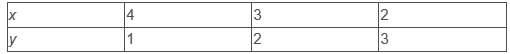And, 2x 2y = 10
x = 10-2y/2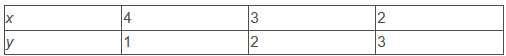Graphical representation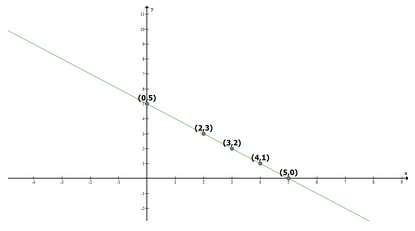From the figure, it can be observed that these lines are overlapping each other. Therefore, infinite solutions are possible for the given pair of equations.

(ii) x – y = 8, 3x – 3y = 16
a1/a2 = 1/3
b1/b2 = -1/-3 = 1/3 and
c1/c2 = 8/16 = 1/2
Hence, a1/a2b1/b c1/c2

Therefore, these linear equations are parallel to each other and thus have no possible solution. Hence, the pair of linear equations is inconsistent.

(iii) 2x   y – 6 = 0, 4x – 2y – 4 = 0
a1/a2 = 2/4 = 1/2
b1/b2 = -1/2 and
c1/c2 = -6/-4 = 3/2
Hence, a1/a2 ≠ b1/b2

Therefore, these linear equations are intersecting each other at one point and thus have only one possible solution. Hence, the pair of linear equations is consistent.

2x y - 6 = 0
y = 6 - 2x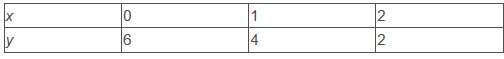And, 4x - 2y -4 = 0
y = 4x - 4/2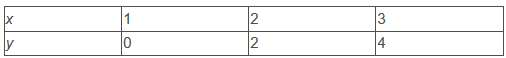Graphical representation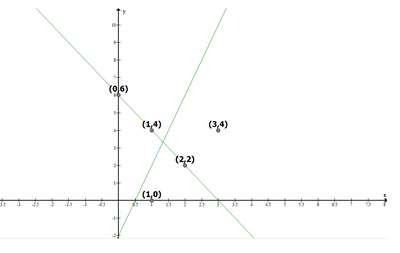From the figure, it can be observed that these lines are intersecting each other at the only one point i.e., (2,2) which is the solution for the given pair of equations.

(iv) 2x – 2y – 2 = 0, 4x – 4y – 5 = 0
a1/a2 = 2/4 = 1/2
b1/b2 = -2/-4 = 1/2 and
c1/c2 = 2/5
Hence, a1/a2b1/b c1/c2

Therefore, these linear equations are parallel to each other and thus, have no possible solution. Hence, the pair of linear equations is inconsistent.

Page No: 50

5. Half the perimeter of a rectangular garden, whose length is 4 m more than its width, is 36 m. Find the dimensions of the garden.

Let length of rectangle = x m
Width of the rectangle = m
According to the question,
y - x = 4 ... (i)
y x = 36 ... (ii)
y - x = 4
y = x 4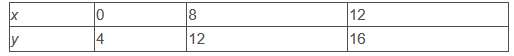y  x = 36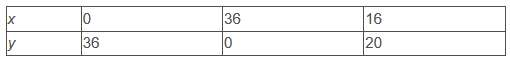Graphical representation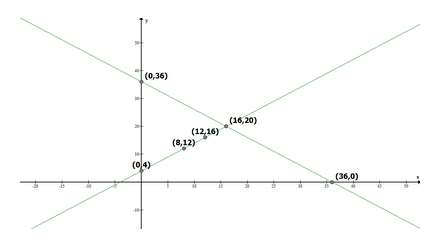From the figure, it can be observed that these lines are intersecting each other at only point i.e., (16, 20). Therefore, the length and width of the given garden is 20 m and 16 m respectively.

6. Given the linear equation 2x 3y - 8 = 0, write another linear equations in two variables such that the geometrical representation of the pair so formed is:
(i) intersecting lines

(ii) parallel lines
(iii) coincident lines

(i) Intersecting lines:
For this condition,
a1/a2 ≠ b1/b2
The second line such that it is intersecting the given line is
2x  4y - 6 = 0 as
a1/a2 = 2/2 = 1
b1/b2 = 3/4 and
a1/a2 ≠ b1/b2

(ii) Parallel lines

For this condition,

a1/a2b1/b c1/c2
Hence, the second line can be
4x  6y - 8 = 0 as
a1/a2 = 2/4 = 1/2
b1/b2 = 3/6 = 1/2 and
c1/c2 = -8/-8 = 1
and a1/a2b1/b c1/c2

(iii) Coincident lines
For coincident lines,
a1/a2b1/b2 = c1/c2
Hence, the second line can be
6x  9y - 24 = 0 as
a1/a2 = 2/6 = 1/3
b1/b2 = 3/9 = 1/3 and
c1/c2 = -8/-24 = 1/3
and a1/a2b1/b2 = c1/c2

7. Draw the graphs of the equations x - y 1 = 0 and 3x 2y - 12 = 0. Determine the coordinates of the vertices of the triangle formed by these lines and the x-axis, and shade the triangular region.

x - y 1 = 0
x = y - 1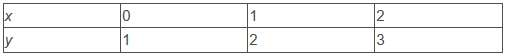3x 2y - 12 = 0

x = 12 - 2y/3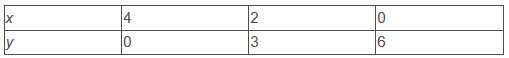Graphical representation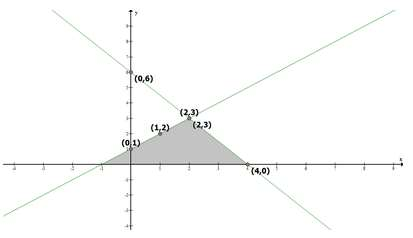From the figure, it can be observed that these lines are intersecting each other at point (2, 3) and x-axis at ( - 1, 0) and (4, 0). Therefore, the vertices of the triangle are (2, 3), ( - 1, 0), and (4, 0).

Page No: 53

Exercise 3.3

1. Solve the following pair of linear equations by the substitution method.
(i) = 14 ; = 4

(ii) = 3 ; s/3 t/2 = 6
(iii) 3xy = 3 ; 9x – 3y = 9

(iv) 0.2x 0.3y = 1.3 ; 0.4x 0.5y = 2.3
(v) √2x √3y = 0 ; √3x - √8y = 0

(vi) 3/2x - 5/3y = -2 ; x/3 y/2 = 13/6

(i) x y = 14 ... (i)

xy = 4 ... (ii)
From equation (i), we get

x = 14 - y ... (iii)
Putting this value in equation (ii), we get

(14 - y) - y = 4

14 - 2y = 4

10 = 2y

= 5 ... (iv)

Putting this in equation (iii), we get

= 9

= 9 and y = 5

(ii) – = 3 ... (i)
s/3  t/2 = 6 ... (ii)
From equation (i), we get= t  3
Putting this value in equation (ii), we get
t 3/3  t/2 = 6
2t  6  3t = 36
5t = 30
t = 30/5 ... (iv)
Putting in equation (iii), we obtain
s = 9
∴ s = 9, t = 6

(iii) 3x - = 3 ... (i)
9x - 3y = 9 ... (ii)
From equation (i), we get
y = 3x - 3 ... (iii)
Putting this value in equation (ii), we get
9x - 3(3x - 3) = 9
9x - 9x  9 = 9
9 = 9
This is always true.
Hence, the given pair of equations has infinite possible solutions and the relation between these variables can be given by
y = 3x - 3
Therefore, one of its possible solutions is x = 1, y = 0.

(iv) 0.2x 0.3y = 1.3 ... (i)
0.4x 0.5y = 2.3 ... (ii)
0.2x 0.3y = 1.3
Solving equation (i), we get
0.2x = 1.3 – 0.3y
Dividing by 0.2, we get
x = 1.3/0.2 - 0.3/0.2
x = 6.5 – 1.5 y(iii)
Putting the value in equation (ii), we get
0.4x 0.5y = 2.3
(6.5 – 1.5y) × 0.4x 0.5y = 2.3
2.6 – 0.6y 0.5y = 2.3
-0.1y = 2.3 – 2.6
= -0.3/-0.1
y = 3
Putting this value in equation (iii) we get
x = 6.5 – 1.5 y
x = 6.5 – 1.5(3)
x = 6.5 - 4.5
x = 2
∴ = 2 and y = 3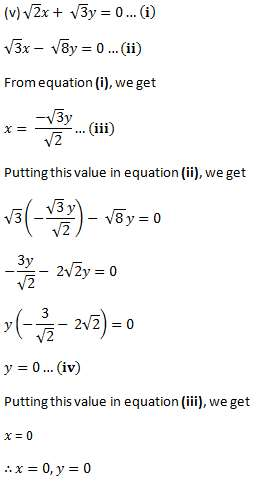(vi) 3/2x - 5/3y = -2 ... (i)

x/3  y/2 = 13/6 ... (ii)

From equation (i), we get

9x - 10y = -12

x = -12  10y/9 ... (iii)

Putting this value in equation (ii), we get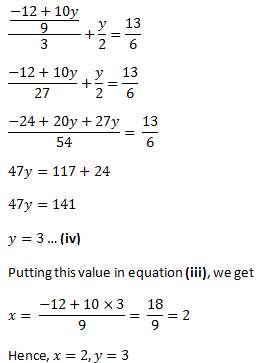2. Solve 2x 3y = 11 and 2x - 4y = - 24 and hence find the value of 'm' for which y =mx 3.

2x 3= 11 ... (i)
Subtracting 3y both side we get
2x = 11 – 3y(ii)
Putting this value in equation second we get
2x – 4y = – 24 … (iii)
11- 3y – 4y = - 24
7y = - 24 – 11
-7y = - 35
y = - 35/-7
y = 5
Putting this value in equation (iii) we get
2x = 11 – 3 × 5
2x = 11- 15
2x = - 4
Dividing by 2 we get
x = - 2
Putting the value of x and y
y = mx 3.
5 = -2m 3
2m = 3 – 5
m = -2/2
m = -1

3. Form the pair of linear equations for the following problems and find their solution by substitution method
(i) The difference between two numbers is 26 and one number is three times the other. Find them.

Let larger number = x
Smaller number = y
The difference between two numbers is 26
xy = 26
x = 26 y
Given that one number is three times the other
So x = 3y
Putting the value of x we get
26y = 3y
-2y = - 2 6
y = 13
So value of x = 3y
Putting value of y, we get
x = 3 × 13 = 39
Hence the numbers are 13 and 39.

(ii) The larger of two supplementary angles exceeds the smaller by 18 degrees. Find them.

Let first angle = x
And second number = y
As both angles are supplementary so that sum will 180
x y = 180
x = 180 - y ... (i)
Difference is 18 degree so that
xy = 18
Putting the value of we get
180 – yy = 18
- 2y = -162
y = -162/-2
y = 81
Putting the value back in equation (i), we get
x = 180 – 81 = 99Hence, the angles are 99º and 81º.

(iii) The coach of a cricket team buys 7 bats and 6 balls for Rs 3800. Later, she buys 3 bats and 5 balls for Rs 1750. Find the cost of each bat and each ball.

Let cost of each bat = Rs x
Cost of each ball = Rs y

Given that coach of a cricket team buys 7 bats and 6 balls for Rs 3800.
7x 6y = 3800
6y = 3800 – 7x
Dividing by 6, we get
y = (3800 – 7x)/6 … (i)

Given that she buys 3 bats and 5 balls for Rs 1750 later.
3x 5= 1750
Putting the value of y
3x 5 ((3800 – 7x)/6) = 1750
Multiplying by 6, we get
18x 19000 – 35x = 10500
-17x =10500 - 19000
-17x = -8500
x = - 8500/- 17
= 500
Putting this value in equation (i) we get
= ( 3800 – 7 × 500)/6
= 300/6
= 50

Hence cost of each bat = Rs 500 and cost of each balls = Rs 50.

(iv) The taxi charges in a city consist of a fixed charge together with the charge for the distance covered. For a distance of 10 km, the charge paid is Rs 105 and for a journey of 15 km, the charge paid is Rs 155. What are the fixed charges and the charge per km? How much does a person have to pay for traveling a distance of 25 km?

Let the fixed charge for taxi = Rs x
And variable cost per km = Rs y
Total cost = fixed charge variable charge
Given that for a distance of 10 km, the charge paid is Rs 105
x 10y = 105 … (i)
x = 105 – 10y
Given that for a journey of 15 km, the charge paid is Rs 155
x 15y = 155
Putting the value of x we get
105 – 10y 15y = 155
5y = 155 – 105
5y = 50
Dividing by 5, we get
y = 50/5 = 10
Putting this value in equation (i) we get
= 105 – 10 × 10
= 5
People have to pay for traveling a distance of 25 km
= x 25y
= 5 25 × 10
= 5 250
=255

A person have to pay Rs 255 for 25 Km.

(v) A fraction becomes 9/11, if 2 is added to both the numerator and the denominator. If, 3 is added to both the numerator and the denominator it becomes 5/6 . Find the fraction.

Let Numerator = x
Denominator = y
Fraction will = x/y
A fraction becomes 9/11, if 2 is added to both the numerator and the denominator
(x 2)/y 2 = 9/11

By Cross multiplication, we get
11x 22 = 9y 18
Subtracting 22 both side, we get
11x = 9y – 4
Dividing by 11, we get
= 9y – 4/11 … (i)
Given that 3 is added to both the numerator and the denominator it becomes 5/6.
If, 3 is added to both the numerator and the denominator it becomes 5/6
(x 3)/ 3  = 5/6 … (ii)
By Cross multiplication, we get
6x 18 = 5y 15
Subtracting the value of x, we get
6(9y – 4 )/11 18 = 5y 15
Subtract 18 both side we get
6(9y – 4 )/11 = 5y - 3
54 – 24 = 55 - 33
-y = -9
y = 9
Putting this value of y in equation (i), we get
= 9y – 4
11 … (i)
x = (81 – 4)/77
x = 77/11
x = 7

Hence our fraction is 7/9.

(vi) Five years hence, the age of Jacob will be three times that of his son. Five years ago, Jacob’s age was seven times that of his son. What are their present ages?

Let present age of Jacob = x year
And present Age of his son is = y year
Five years hence,
Age of Jacob will = x 5 year
Age of his son will = y 5year
Given that the age of Jacob will be three times that of his son
x 5 = 3( 5)
Adding 5 both side, we get
x = 3y 15 - 5
x = 3y 10 … (i)
Five years ago,
Age of Jacob will = x - 5 year
Age of his son will = y - 5 year
Jacob’s age was seven times that of his son
x – 5 = 7(y -5)
Putting the value of x from equation (i) we get
3 10 – 5 = 7y – 35
3y 5 = 7y – 35
3y – 7y = -35 – 5
-4y = - 40
y = - 40/- 4
y = 10 year
Putting the value of y in equation first we get
x = 3 × 10 10
x = 40 years
Hence, Present age of Jacob = 40 years and present age of his son = 10 years.

Page No: 56

Exercise 3.4

1. Solve the following pair of linear equations by the elimination method and the substitution method:
(i) x y =5 and 2x –3y = 4
(ii) 3x 4y = 10 and 2x – 2y = 2
(iii) 3x – 5y – 4 = 0 and 9x = 2y 7
(iv) x/2 2y/3 = - 1 and xy/3 = 3

(i) x   y =5 and 2x –3y = 4
By elimination method
x y =5 ... (i)
2x –3y = 4 ... (ii)
Multiplying equation (i) by (ii), we get
2x 2y = 10 ... (iii)
2x –3y = 4 ... (ii)
Subtracting equation (ii) from equation (iii), we get
5y = 6
y = 6/5
Putting the value in equation (i), we get
x = 5 - (6/5) = 19/5

Hence, x = 19/5 and y = 6/5

By substitution methodx y = 5 ... (i)
Subtracting y both side, we get
x = 5 - y ... (iv)
Putting the value of x in equation (ii) we get
2(5 – y) – 3y = 4
-5y = - 6
y = -6/-5 = 6/5
Putting the value of y in equation (iv) we get
x = 5 – 6/5
x = 19/5
Hence, x = 19/5 and y = 6/5 again

(ii) 3x  4y = 10 and 2x – 2y = 2
By elimination method
3x 4y = 10 .... (i)
2x – 2y = 2 ... (ii)
Multiplying equation (ii) by 2, we get
4x – 4y = 4 ... (iii)
3x 4y = 10 ... (i)
Adding equation (i) and (iii), we get
7x 0 = 14
Dividing both side by 7, we get
x = 14/7 = 2
Putting in equation (i), we get
3x 4y = 10
3(2) 4y = 10
6 4y = 10
4y = 10 – 6
4y = 4
y = 4/4 = 1

Hence, answer is x = 2, y = 1

By substitution method
3x 4y = 10 ... (i)
Subtract 3x both side, we get
4y = 10 – 3x
Divide by 4 we get
y = (10 - 3x )/4
Putting this value in equation (ii), we get
2x – 2y = 2 ... (i)
2x – 2(10 - 3x )/4) = 2
Multiply by 4 we get
8x - 2(10 – 3x) = 8
8x - 20 6x = 8
14x = 28
x = 28/14 = 2
= (10 - 3x)/4

= 4/4 = 1

Hence, answer is x = 2, y = 1 again.

(iii) 3x – 5y – 4 = 0 and 9x = 2y 7
By elimination method
3x – 5y – 4 = 0
3x – 5y = 4 ...(i)
9x = 2y 7
9x – 2 = 7 ... (ii)
Multiplying equation (i) by 3, we get
9 x – 15 y = 11 ... (iii)
9x – 2y = 7 ... (ii)
Subtracting equation (ii) from equation (iii), we get
-13y = 5
y = -5/13
Putting value in equation (i), we get
3x – 5y = 4 ... (i)
3x - 5(-5/13) = 4
Multiplying by 13 we get
39x 25 = 52
39x = 27
x =27/39 = 9/13
Hence our answer is x = 9/13 and y = - 5/13

By substitution method
3x – 5y = 4 ... (i)
Adding 5y both side we get
3x = 4 5y
Dividing by 3 we get
x = (4 5y )/3 ... (iv)
Putting this value in equation (ii) we get
9x – 2y = 7 ... (ii)
9 ((4 5)/3) – 2y = 7
Solve it we get
3(4 5y ) – 2y = 7
12 15y – 2y = 7
13y = - 5
y = -5/13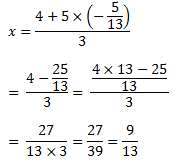Hence we get x = 9/13 and y = - 5/13 again.

(iv) x/2 2y/3 = - 1 and x – y/3 = 3
By elimination method
x/2 2y/3 = -1 ... (i)
xy/3 = 3 ... (ii)
Multiplying equation (i) by 2, we get
x 4y/3 = - 2 ... (iii)
xy/3 = 3 ... (ii)
Subtracting equation (ii) from equation (iii), we get
5y/3 = -5
Dividing by 5 and multiplying by 3, we get
= -15/5
= - 3
Putting this value in equation (ii), we get
xy/3 = 3 ... (ii)
x – (-3)/3 = 3
x 1 = 3
x = 2

Hence our answer is x = 2 and y = −3.

By substitution method
xy/3 = 3 ... (ii)
Add y/3 both side, we get
= 3 y/3 ... (iv)
Putting this value in equation (i) we get
x/2 2y/3 = - 1 ... (i)
(3 y/3)/2 2y/3 = -1
3/2 y/6 2y/3 = - 1
Multiplying by 6, we get
9 y 4 = - 6
5y = -15
y = - 3

Hence our answer is x = 2 and y = −3.

Page No: 57

2. Form the pair of linear equations in the following problems, and find their solutions (if they exist) by the elimination method:

(i) If we add 1 to the numerator and subtract 1 from the denominator, a fraction reduces to 1. It becomes 1/2 if we only add 1 to the denominator. What is the fraction?

(ii) Five years ago, Nuri was thrice as old as Sonu. Ten years later, Nuri will be twice as old as Sonu. How old are Nuri and Sonu?

(iii) The sum of the digits of a two-digit number is 9. Also, nine times this number is twice the number obtained by reversing the order of the digits. Find the number.

(iv) Meena went to bank to withdraw Rs 2000. She asked the cashier to give her Rs 50 and Rs 100 notes only. Meena got 25 notes in all. Find how many notes of Rs 50 and Rs 100 she received.

(v) A lending library has a fixed charge for the first three days and an additional charge for each day thereafter. Saritha paid Rs 27 for a book kept for seven days, while Susy paid Rs 21 for the book she kept for five days. Find the fixed charge and the charge for each extra day.

(i) Let the fraction be x/y
According to the question,x  1/y - 1 = 1
- = -2 ... (i)x/y 1 = 1/2
⇒ 2x - = 1 ... (ii)
Subtracting equation (i) from equation (ii), we get
x = 3 ... (iii)
Putting this value in equation (i), we get
3 - y = -2
-y = -5
y = 5
Hence, the fraction is 3/5

(ii) Let present age of Nuri = x
and present age of Sonu = y
According to the given information,question,(x - 5) = 3(y - 5)
x - 3y = -10 ... (i)
(x  10y) = 2(y 10)
x - 2y = 10 ... (ii)
Subtracting equation (i) from equation (ii), we get
y = 20 ... (iii)
Putting this value in equation (i), we get
x - 60 = -10
= 50
Hence, age of Nuri = 50 years and age of Sonu = 20 years.

(iii) Let the unit digit and tens digits of the number be and respectively.
Then, number = 10y x
Number after reversing the digits = 10x y
According to the question,
x y = 9 ... (i)
9(10 x) = 2(10x y)
88y - 11x = 0
- x 8y =0 ... (ii)
Adding equation (i) and (ii), we get
9y = 9
y = 1 ... (iii)
Putting the value in equation (i), we get
x = 8
Hence, the number is 10y  x = 10 × 1 8 = 18.

(iv) Let the number of Rs 50 notes and Rs 100 notes be x and y respectively.
According to the question,
= 25 ... (i)
50x  100y = 2000 ... (ii)
Multiplying equation (i) by 50, we get
50x  50y = 1250 ... (iii)
Subtracting equation (iii) from equation (ii), we get
50y = 750
y = 15
Putting this value in equation (i), we have x = 10
Hence, Meena has 10 notes of Rs 50 and 15 notes of Rs 100.

(v) Let the fixed charge for first three days and each day charge thereafter be Rs and Rs respectively.
According to the question,
4y = 27 ... (i)
2y = 21 ... (ii)
Subtracting equation (ii) from equation (i), we get
2y = 6
y = 3 ... (iii)
Putting in equation (i), we get
x  12 =27
x = 15
Hence, fixed charge = Rs 15 and Charge per day = Rs 3.

Page No: 62

Exercise 3.5

1. Which of the following pairs of linear equations has unique solution, no solution or infinitely many solutions? In case there is a unique solution, find it by using cross multiplication method.

(i) x – 3y – 3 = 0 ; 3x – 9y – 2 =0
(ii) 2x y = 5 ; 3x 2y =8
(iii) 3x – 5y = 20 ; 6x – 10y =40
(iv) x – 3y – 7 = 0 ; 3x – 3y – 15= 0

(i) x – 3y – 3 = 0
3x – 9y – 2 =0
a1/a2 = 1/3
b1/b2 = -3/-9 = 1/3 and
c1/c2 = -3/-2 = 3/2
a1/a2b1/b c1/c2

Therefore, the given sets of lines are parallel to each other. Therefore, they will not intersect each other and thus, there will not be any solution for these equations.

(ii) 2x   y = 5
3x  2y = 8
a1/a2 = 2/3
b1/b2 = 1/2 and
c1/c2 = -5/-8 = 5/8
a1/a2 b1/b2

Therefore, they will intersect each other at a unique point and thus, there will be a unique solution for these equations.

By cross-multiplication method,
x/b1c2-b2c= y/c1a2-c2a= 1/a1b2-a2b1
x/-8-(-10) = y/-15 16 = 1/4-3
x/2 = y/1 = 1
x/2 = 1, y/1 = 1
= 2, = 1.

(iii) 3x – 5y = 20
6x – 10y = 40
a1/a2 = 3/6 = 1/2
b1/b2 = -5/-10 = 1/2 and
c1/c2 = -20/-40 = 1/2
a1/a2 = b1/b= c1/c2

Therefore, the given sets of lines will be overlapping each other i.e., the lines will be coincident to each other and thus, there are infinite solutions possible for these equations.

(iv) x – 3y – 7 = 0
3x – 3y – 15= 0
a1/a2 = 1/3
b1/b2 = -3/-3 = 1 and
c1/c2 = -7/-15 = 7/15
a1/a2 b1/b2

Therefore, they will intersect each other at a unique point and thus, there will be a unique solution for these equations.

By cross-multiplication,

x/45-(21) = y/-21-(-15) = 1/-3-(-9)
x/24 = y/-6 = 1/6
x/24 = 1/6 and y/-6 = 1/6
= 4 and = -1
∴ = 4, = -1.

2. (i) For which values of a and b does the following pair of linear equations have an infinite number of solutions?
2x 3y =7

(ab)x (a  b)y = 3a b –2

2x  3y -7 = 0

(a – b)x  (a   b)y - (3a  b –2) = 0

a1/a2 = 2/a-b = 1/2
b1/b2 = -7/a b and
c1/c2 = -7/-(3a b-2) = 7/(3a b-2)
For infinitely many solutions,a1/a2b1/b2 = c1/c2

2/a-= 7/3a b-26a 2b - 4 = 7a - 7b
a - 9b = -4 ... (i)

2/a-= 3/a b
2a  2b = 3a - 3b
a - 5b = 0 ... (ii)

Subtracting equation (i) from (ii), we get
4b = 4
b = 1
Putting this value in equation (ii), we get
a - 5 × 1 = 0
a = 5
Hence, a = 5 and b = 1 are the values for which the given equations give infinitely many solutions.

(ii) For which value of k will the following pair of linear equations have no solution?

3x = 1

(2k –1)x (k –1)y = 2k 1

3x   -1 = 0

(2k –1)x  (k –1)y - (2k  1) = 0

a1/a2 = 3/2k-1
b1/b2 = 1/k-1 and
c1/c2 = -1/-2k-1 = 1/2k 1
For no solutions,
a1/a2b1/b c1/c2
3/2k-1 = 1/k-1 ≠ 1/2k 1

3/2k-1 = 1/k-1
3k - 3 = 2k - 1
k = 2
Hence, for k = 2, the given equation has no solution.

3. Solve the following pair of linear equations by the substitution and cross-multiplication methods:
8x 5y = 9
3x 2y = 4

8x  5y = 9 ... (i)
3x  2y = 4 ... (ii)
From equation (ii), we get
x = 4-2y/3 ... (iii)
Putting this value in equation (i), we get
8(4-2y/3)  5y = 9
32 - 16y  15y = 27
-y = -5
y = 5 ... (iv)
Putting this value in equation (ii), we get
3x  10 = 4
x = -2
Hence, x = -2, = 5
By cross multiplication again, we get

8x  5y -9 = 0

3x  2y - 4 = 0

x/-20-(-18) = y/-27-(-32) = 1/16-15
x/-2 = y/5 = 1/1
x/-2 = 1 and y/5 = 1
x = -2 and y = 5

4. Form the pair of linear equations in the following problems and find their solutions (if they exist) by any algebraic method:

(i) A part of monthly hostel charges is fixed and the remaining depends on the number of days one has taken food in the mess. When a student A takes food for 20 days she has to pay Rs 1000 as hostel charges whereas a student B, who takes food for 26 days, pays Rs 1180 as hostel charges. Find the fixed charges and the cost of food per day.

Let be the fixed charge of the food and be the charge for food per day.

According to the question,

x  20y = 1000 ... (i)

x  26y = 1180 ... (ii)
Subtracting equation (i) from equation (ii), we get

6y = 180

y = 180/6 = 30
Putting this value in equation (i), we get
x  20 × 30 = 1000
x = 1000 - 600
x = 400
Hence, fixed charge = Rs 400 and charge per day = Rs 30

(ii) A fraction becomes 1/3 when 1 is subtracted from the numerator and it becomes 1/4 when 8 is added to its denominator. Find the fraction.

Let the fraction be x/y
According to the question,

x-1/y = 1/3
⇒ 3x - y = 3... (i)
x/y 8 = 1/4
⇒ 4x - y = 8 ... (ii)
Subtracting equation (i) from equation (ii), we get

x = 5 ... (iii)

Putting this value in equation (i), we get

15 - = 3

= 12
Hence, the fraction is 5/12.

(iii) Yash scored 40 marks in a test, getting 3 marks for each right answer and losing 1 mark for each wrong answer. Had 4 marks been awarded for each correct answer and 2 marks been deducted for each incorrect answer, then Yash would have scored 50 marks. How many questions were there in the test?

Let the number of right answers and wrong answers be and respectively.

According to the question,

3x - = 40 ... (i)

4x - 2y = 50

⇒ 2x - = 25 ... (ii)

Subtracting equation (ii) from equation (i), we get
= 15 ... (iii)
Putting this value in equation (ii), we get

30 - = 25

= 5

Therefore, number of right answers = 15
And number of wrong answers = 5
Total number of questions = 20

(iv) Places A and B are 100 km apart on a highway. One car starts from A and another from B at the same time. If the cars travel in the same direction at different speeds, they meet in 5 hours. If they travel towards each other, they meet in 1 hour. What are the speeds of the two cars?

Let the speed of 1st car and 2nd car be u km/h and v km/h.
Respective speed of both cars while they are travelling in same direction = (u - v) km/h

Respective speed of both cars while they are travelling in opposite directions i.e., travelling towards each other = ( v) km/h

According to the question,

5(- v) = 100

u - v = 20 ... (i)

1(u  v) = 100 ... (ii)

Adding both the equations, we get

2u = 120

u = 60 km/h ... (iii)

Putting this value in equation (ii), we obtain
v = 40 km/h
Hence, speed of one car = 60 km/h and speed of other car = 40 km/h

(v) The area of a rectangle gets reduced by 9 square units, if its length is reduced by 5 units and breadth is increased by 3 units. If we increase the length by 3 units and the breadth by 2 units, the area increases by 67 square units. Find the dimensions of the rectangle.

Let length and breadth of rectangle be unit and y unit respectively.
Area = xy
According to the question,
(x - 5) (y  3) = xy - 9
⇒ 3x - 5y - 6 = 0 ... (i)
(x  3) (y  2) = xy  67
⇒ 2x - 3y - 61 = 0 ... (ii)
By cross multiplication, we get
x/305-(-18) = y/-12-(-183) = 1/9-(-10)
x/323 = y/171 = 1/19
= 17, y = 9
Hence, the length of the rectangle = 17 units and breadth of the rectangle = 9 units.

Page No: 67

Exercise 3.6

1. Solve the following pairs of equations by reducing them to a pair of linear equations:
(i) 1/2x  1/3y = 2
1/3x  1/2= 13/6

(ii) 2/√x 3/√y = 2
4/√x - 9/√y = -1

(iii) 4/x  3y = 14
3/x - 4y = 23

(iv) 5/x-1  1/y-2 = 2
6/x-1 - 3/y-2 = 1

(v) 7x-2y/xy = 5
8x  7y/xy = 15

(vi) 6x  3y = 6xy
2x  4y = 5xy

(vii) 10/x y  2/x-y = 4
15/x y - 5/x-y = -2

(viii) 1/3x y  1/3x-y = 3/4
1/2(3x-y) - 1/2(3x-y) = -1/8

(i) 1/2x  1/3y = 2
1/3x  1/2= 13/6
Let 1/= p and 1/= q, then the equations changes as below:
p/2  q/3 = 2
⇒ 3p  2q -12 = 0 ... (i)
p/3  q/2 = 13/6
⇒ 2p  3q -13 = 0 ... (ii)

By cross-multiplication method, we get

p/-26-(-36) = q/-24-(-39) = 1/9-4
p/10 = q/15 = 1/5
p/10 = 1/5 and q/15 = 1/5
= 2 and = 3
1/= 2 and 1/= 3
Hence, = 1/2 and = 1/3

(ii) 2/√x  3/√y = 2
4/√x - 9/√y = -1
Let 1/√= p and 1/√y = q, then the equations changes as below:
2p  3q = 2 ... (i)
4p - 9q = -1 ... (ii)
Multiplying equation (i) by 3, we get
6p 9q = 6 ... (iii)
Adding equation (ii) and (iii), we get

10p = 5

p = 1/2 ... (iv)

Putting in equation (i), we get

2 × 1/2  3q = 2
3q = 1
q = 1/3

= 1/√x = 1/2
x = 2
x = 4
and
= 1/√y = 1/3
y = 3
y = 9
Hence, x = 4, y = 9

(iii) 4/x  3y = 14
3/x - 4y = 23
Putting 1/x = p in the given equations, we get
4p  3y = 14
⇒ 4p  3y - 14 = 0
3p - 4y = 23
⇒ 3p - 4y -23 = 0
By cross-multiplication, we get
p/-69-56 = y/-42-(-92) = 1/-16-9
⇒ -p/125 = y/50 = -1/25
Now,
-p/125 = -1/25 and y/50 = -1/25
p = 5 and y = -2
Also, p = 1/x = 5
x = 1/5
So, x = 1/5 and y = -2 is the solution.

(iv) 5/x-1  1/y-2 = 2
6/x-1 - 3/y-2 = 1
Putting 1/x-1 = p and 1/y-2 = q in the given equations, we obtain
5p  q = 2 ... (i)
6p - 3q = 1 ... (ii)
Now, by multiplying equation (i) by 3 we get
15p  3q = 6 ... (iii)
Now, adding equation (ii) and (iii)
21p = 7
p = 1/3
Putting this value in equation (ii) we get,
6×1/3 - 3q =1
⇒ 2-3q = 1
⇒ -3q = 1-2
⇒ -3q = -1
⇒ q = 1/3
Now,
p = 1/x-1 = 1/3
⇒1/x-1 = 1/3
⇒ 3 = - 1
⇒ x = 4
Also,
q = 1/y-2 = 1/3
⇒ 1/y-2 = 1/3
⇒ 3 = y-2
⇒ y = 5
Hence, x = 4 and y = 5 is the solution.

(v) 7x-2y/xy = 5
⇒ 7x/xy - 2y/xy = 5
⇒ 7/y - 2/x = 5 ... (i)
8x 7y/xy = 15
⇒ 8x/xy  7y/xy = 15
⇒ 8/y  7/x = 15 ... (ii)
Putting 1/x = p and 1/y = q in (i) and (ii) we get,
7q - 2p = 5 ... (iii)
8q  7p = 15 ... (iv)
Multiplying equation (iii) by 7 and multiplying equation (iv) by 2 we get,
49q - 14p = 35 ... (v)
16q  14p = 30 ... (vi)
Now, adding equation (v) and (vi) we get,
49q - 14p   16q  14p = 35  30
⇒ 65q = 65
q = 1
Putting the value of q in equation (iv)
8  7p = 15
⇒ 7p = 7
p = 1
Now,
p = 1/x = 1
⇒ 1/x = 1
x = 1
also, q = 1 = 1/y
⇒ 1/y = 1
= 1
Hence, =1 and y = 1 is the solution.

(vi) 6x  3y = 6xy
⇒ 6x/xy  3y/xy = 6
⇒ 6/y  3/x = 6 ... (i)
2x  4y = 5xy
⇒ 2x/xy  4y/xy = 5
⇒ 2/y  4/x = 5 ... (ii)
Putting 1/x = p and 1/y = q in (i) and (ii) we get,
6q  3p - 6 = 0
2q  4p - 5 = 0
By cross multiplication method, we get
p/-30-(-12) = q/-24-(-15) = 1/6-24
p/-18 = q/-9 = 1/-18
p/-18 = 1/-18 and q/-9 = 1/-18
= 1 and q = 1/2
= 1/x = 1 and q = 1/y = 1/2
= 1, y = 2
Hence, = 1 and = 2

(vii) 10/x y  2/x-y = 4
15/x y - 5/x-y = -2
Putting 1/x y = p and 1/x-y = q in the given equations, we get:
10p  2q = 4
⇒ 10p  2q - 4 = 0 ... (i)
15p - 5q = -2
⇒ 15p - 5q  2 = 0 ... (ii)
Using cross multiplication, we get
p/4-20 = q/-60-(-20) = 1/-50-30
p/-16 = q/-80 = 1/-80
p/-16 = 1/-80 and q/-80 = 1/-80
p = 1/5 and q = 1
p = 1/x y = 1/5 and q = 1/x-y = 1
x  y = 5 ... (iii)
and x - y = 1 ... (iv)
Adding equation (iii) and (iv), we get
2x = 6
x = 3 .... (v)
Putting value of x in equation (iii), we get
y = 2
Hence, x = 3 and y = 2

(viii) 1/3x y  1/3x-y = 3/4
1/2(3x-y) - 1/2(3x-y) = -1/8
Putting 1/3x y = p and 1/3x-y = q in the given equations, we get
p  q = 3/4 ... (i)
p/2 - q/2 = -1/8
- = -1/4 ... (ii)
Adding (i) and (ii), we get
2p = 3/4 - 1/4
2p = 1/2
= 1/4
Putting the value in equation (ii), we get
1/4 - q = -1/4
q = 1/4  1/4 = 1/2
p = 1/3x y = 1/4
3x  y = 4 ... (iii)
q = 1/3x-y = 1/2
3xy = 2 ... (iv)
Adding equations (iii) and (iv), we get
6x = 6
x = 1 ... (v)
Putting the value in equation (iii), we get
3(1)  y = 4
y = 1
Hence, x = 1 and y = 1

2. Formulate the following problems as a pair of equations, and hence find their solutions:

(i) Ritu can row downstream 20 km in 2 hours, and upstream 4 km in 2 hours. Find her speed of rowing in still water and the speed of the current.

Let the speed of Ritu in still water and the speed of stream be x km/h
and y km/h respectively.
Speed of Ritu while rowing
Upstream = (x - y) km/h

Downstream = (x  y) km/h

According to question,

2(x  y) = 20

x  y = 10 ... (i)
2(x - y) = 4
x - y = 2 ... (ii)
Adding equation (i) and (ii), we get

Putting this equation in (i), we get

y = 4

Hence, Ritu's speed in still water is 6 km/h and the speed of the current is 4 km/h.

(ii) 2 women and 5 men can together finish an embroidery work in 4 days, while 3 women and 6 men can finish it in 3 days. Find the time taken by 1 woman alone to finish the work, and also that taken by 1 man alone.

Let the number of days taken by a woman and a man be x and y respectively.
Therefore, work done by a woman in 1 day = 1/x

According to the question,

4(2/x  5/y) = 1

2/x  5/y = 1/4

3(3/x  6/y) = 1

3/x  6/y = 1/3

Putting 1/x = p and 1/= q in these equations, we get

2p  5q = 1/4

By cross multiplication, we get

p/-20-(-18) = q/-9-(-18) = 1/144-180

p/-2 = q/-1 = 1/-36

p/-2 = -1/36 and q/-1 = 1/-36

= 1/18 and q = 1/36

p = 1/x = 1/18 and q = 1/y = 1/36

= 18 and = 36

Hence, number of days taken by a woman = 18 and number of days taken by a man = 36

(iii) Roohi travels 300 km to her home partly by train and partly by bus. She takes 4 hours if she travels 60 km by train and remaining by bus. If she travels 100 km by train and the remaining by bus, she takes 10 minutes longer. Find the speed of the train and the bus separately.

Let the speed of train and bus be u km/h and v km/h respectively.
According to the given information,

60/u  240/= 4 ... (i)
100/u  200/= 25/6 ... (ii)
Putting 1/u = p and 1/v = q in the equations, we get
60p  240q = 4 ... (iii)
100p  200q = 25/6
600p  1200q = 25 ... (iv)
Multiplying equation (iii) by 10, we get
600p  2400q = 40 .... (v)
Subtracting equation (iv) from (v), we get1200q = 15
q = 15/200 = 1/80 ... (vi)
Putting equation (iii), we get
60p  3 = 4
60p = 1
p = 1/60
p = 1/u = 1/60 and q = 1/v = 1/80
u = 60 and v = 80
Hence, speed of train = 60 km/h and speed of bus = 80 km/h.

The document NCERT Solutions, Pair Of Linear Equations In Two Variables, Class 10, Maths Notes - Class 10 is a part of Class 10 category.
All you need of Class 10 at this link: Class 10Use Code STAYHOME200 and get INR 200 additional OFF Use Coupon Code

Top Courses for Class 10Top Courses for Class 10Track your progress, build streaks, highlight & save important lessons and more!

,

,

,

,

,

,

,

,

,

,

,

,

,

,

,

,

,

,

,

,

,

,

,

,

,

,

,

,

,

,

;